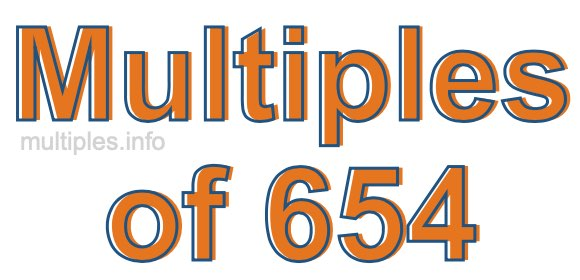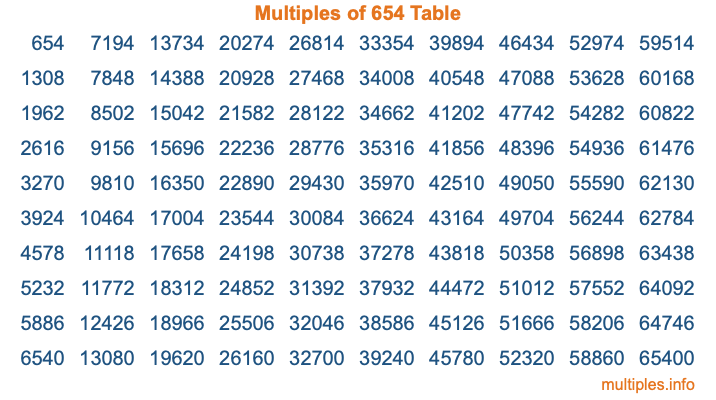Multiples of 654Welcome to the Multiples of 654 page. Here we will first teach you everything you will ever need to know about the multiples of 654, and then give you a study guide summary of everything we taught you to make sure you remember it all. Use this page to look up facts and learn information about the multiples of 654. This page will make you a multiples of six hundred fifty-four expert!

Definition of Multiples of 654
Multiples of 654 are all the numbers that when divided by 654 equal an integer. Each of the multiples of 654 are called a multiple. A multiple of 654 is created by multiplying 654 by an integer.

Therefore, to create a list of multiples of 654, you start with 1 multiplied by 654, then 2 multiplied by 654, then 3 multiplied by 654, and so on for as long as you want. Thus, the list of the first five multiples of 654 is 654, 1308, 1962, 2616, and 3270. To see a larger list of multiples of 654, see the printable image of Multiples of 654 further down on this page. We also have a category where you can choose any nth multiple of 654.

Multiples of 654 Checker
The Multiples of 654 Checker below checks to see if any number of your choice is a multiple of 654. In other words, it checks to see if there is any number (integer) that when multiplied by 654 will equal your number. To do that, we divide your number by 654. If the the quotient is an integer, then your number is a multiple of 654.

Is  a multiple of 654?

Least Common Multiple of 654 and ...
A Least Common Multiple (LCM) is the lowest multiple that two or more numbers have in common. This is also called the smallest common multiple or lowest common multiple and is useful to know when you are adding our subtracting fractions. Enter one or more numbers below (654 is already entered) to find the LCM.

Check out our LCM Calculator if you need more details about the Least Common Multiple or if you need the LCM for different numbers for adding and subtraction fractions.

nth Multiple of 654
As we stated above, 654 is the first multiple of 654, 1308 is the second multiple of 654, 1962 is the third multiple of 654, and so on. Enter a number below to find the nth multiple of 654.

th multiple of 654

Multiples of 654 vs Factors of 654
654 is a multiple of 654 and a factor of 654, but that is where the similarities end. All postive multiples of 654 are 654 or greater than 654. All positive factors of 654 are 654 or less than 654.

Below is the beginning list of multiples of 654 and the factors of 654 so you can compare:

Multiples of 654: 654, 1308, 1962, 2616, 3270, etc.

Factors of 654: 1, 2, 3, 6, 109, 218, 327, 654

As you can see, the multiples of 654 are all the numbers that you can divide by 654 to get a whole number. The factors of 654, on the other hand, are all the whole numbers that you can multiply by another whole number to get 654.

It's also interesting to note that if a number (x) is a factor of 654, then 654 will also be a multiple of that number (x).

Multiples of 654 vs Divisors of 654
The divisors of 654 are all the integers that 654 can be divided by evenly. Below is a list of the divisors of 654.

Divisors of 654: 1, 2, 3, 6, 109, 218, 327, 654

The interesting thing to note here is that if you take any multiple of 654 and divide it by a divisor of 654, you will see that the quotient is an integer.

Multiples of 654 Table
Below is an image of the first 100 multiples of 654 in a table. The table is in chronological order, column by column. The first column has the first ten multiples of 654, the second column has the next ten multiples of 654, and so on.The Multiples of 654 Table is also referred to as the 654 Times Table or Times Table of 654. You are welcome to print out our table for your studies.

Negative Multiples of 654
Although not often discussed or needed in math, it is worth mentioning that you can make a list of negative multiples of 654 by multiplying 654 by -1, then by -2, then by -3, and so on, to get the following list of negative multiples of 654:

-654, -1308, -1962, -2616, -3270, etc.

Multiples of 654 Summary
Below is a summary of important Multiples of 654 facts that we have discussed on this page. To retain the knowledge on this page, we recommend that you read through the summary and explain to yourself or a study partner why they hold true.

There are an infinite number of multiples of 654.

A multiple of 654 divided by 654 will equal a whole number.

654 divided by a factor of 654 equals a divisor of 654.

The nth multiple of 654 is n times 654.

The largest factor of 654 is equal to the first positive multiple of 654.

654 is a multiple of every factor of 654.

654 is a multiple of 654.

A multiple of 654 divided by a divisor of 654 equals an integer.

654 divided by a divisor of 654 equals a factor of 654.

Any integer times 654 will equal a multiple of 654.

Multiples of a Number
Here you can get the multiples of another number, all with the same attention to detail as we did for multiples of 654 on this page.

Multiples of
Multiples of 655
Did you find our page about multiples of six hundred fifty-four educational? Do you want more knowledge? Check out the multiples of the next number on our list!